"MathStudio" App Analytics: ASO Keyword Monitoring | ASOTools

# "MathStudio" App Analytics: ASO Keyword Monitoring | ASOTools

Are you doing MathStudio app analytics for better app store ASO strategies? Whether MathStudio in app store keep on top? What keyword tactics MathStudio take to rank well in app store? Are app store keywords like "mathstudio", "matlab graphing calculator", "math studio" matter in MathStudio ranking?

Free ASO tools for Google Play & App Store, ASOTools will reveal the app store keywords secrets of MathStudio.

## App Analytics: MathStudio

MathStudio-Symbolic graphing calculator, published by Pomegranate Apps LLC in app store, released on 2015-02-18, and last updated 2020-04-04. It belongs to Education category, and Education category is ranking 189 in app store. Currently, MathStudio has 135 ratings, the average rating is 4. Additionally, it had - downloads and - revenue last month in app store worldwide, and the daily active users is -.

More MathStudio info and other app analytics can be found freely in ASOTool.

## ASO Keyword Monitoring: MathStudio

### 1.MathStudio Top 10 App Store Keywords

 Keyword Search Volume KD Related Apps MathStudio's Rank mathstudio 6 4605 30 207 1 matlab graphing calculator - - 21 6 1 math studio - - 51 229 1 pomegranate apps llc 2 1068 11 20 1 ارائه مطلب - - 10 66 1 مطلب الشفاء - - 10 69 1 matlab mobile application - - 10 28 1 matlab calculator - - 10 28 1 matlab application - - 10 28 1 matlab tutorial 2 - 10 29 1

App store keywords matter for ASO. If your app is similar to MathStudio, when you optimize app store keyword ranking, pay attention to the metrics above. ASOTools will show you all of them for free.

Obviously, MathStudio is ranking for "mathstudio", "matlab graphing calculator", "math studio", "pomegranate apps llc", "ارائه مطلب", "مطلب الشفاء", "matlab mobile application", "matlab calculator", "matlab application", and "matlab tutorial" these top 10 keywords in app store.

Also, you'll find MathStudio's position in app store search result. MathStudio is ranking 1 for mathstudio, ranking 1 for matlab graphing calculator, ranking 1 for math studio, and ranking 1 for pomegranate apps llc. For more MathStudio keyword monitoring, please visit asotools.io.

### 2. Top 5 Keyword-Related Apps& App Analytics

• mathstudio  Related Apps & App Analytics
 Apps Average Star Ratings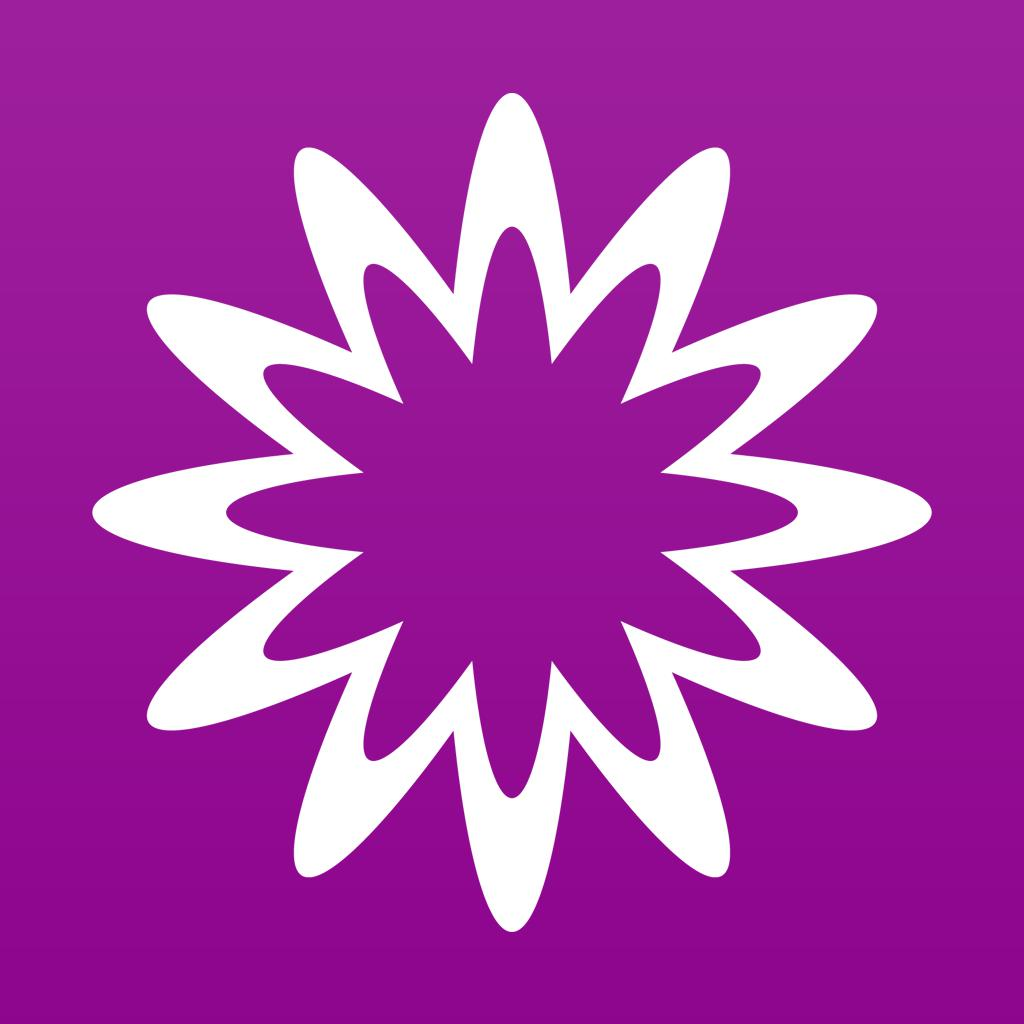MathStudio-Symbolic graphing calculator 4 135Mathway: Math Problem Solver-Homework scanner & calculator 5 309194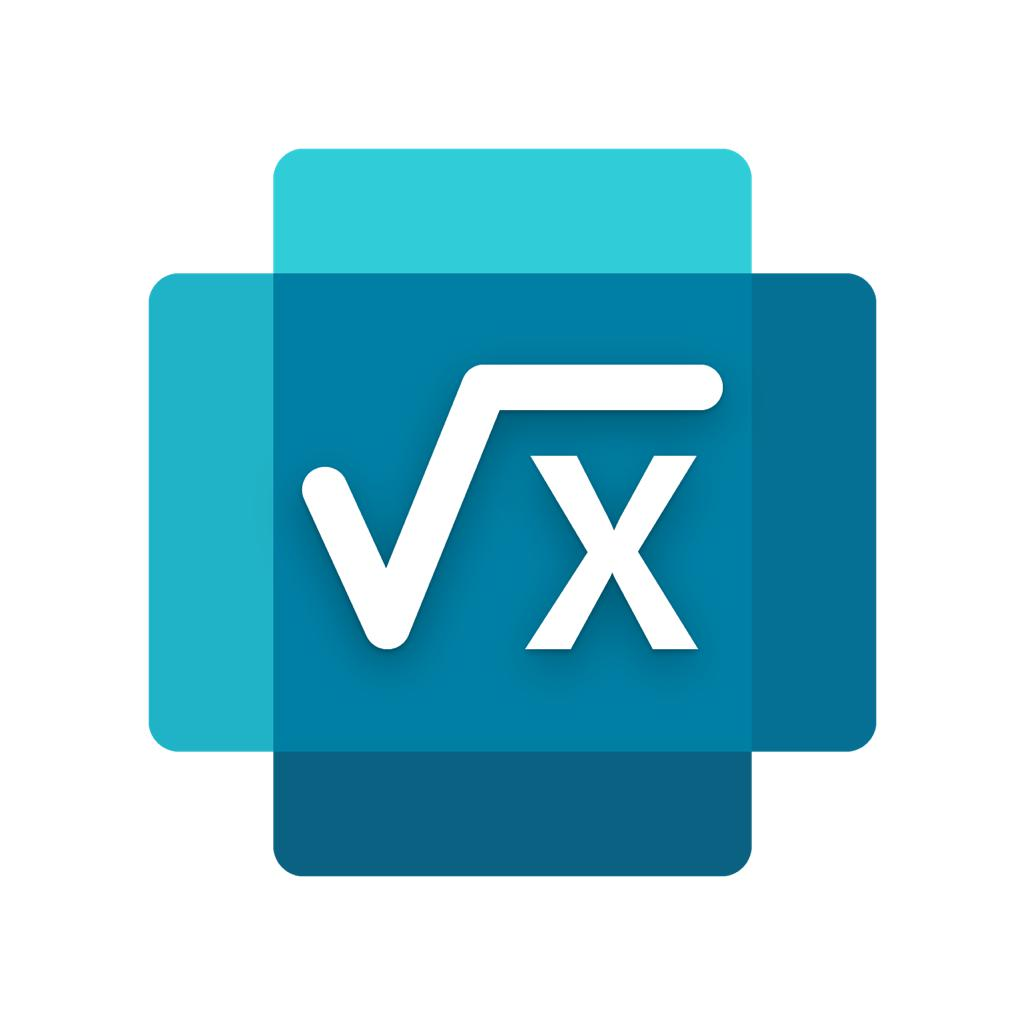Microsoft Math Solver - HW app-Math Homework Scanner & Tutor 5 20565MathStudio Express-Symbolic graphing calculator 4 84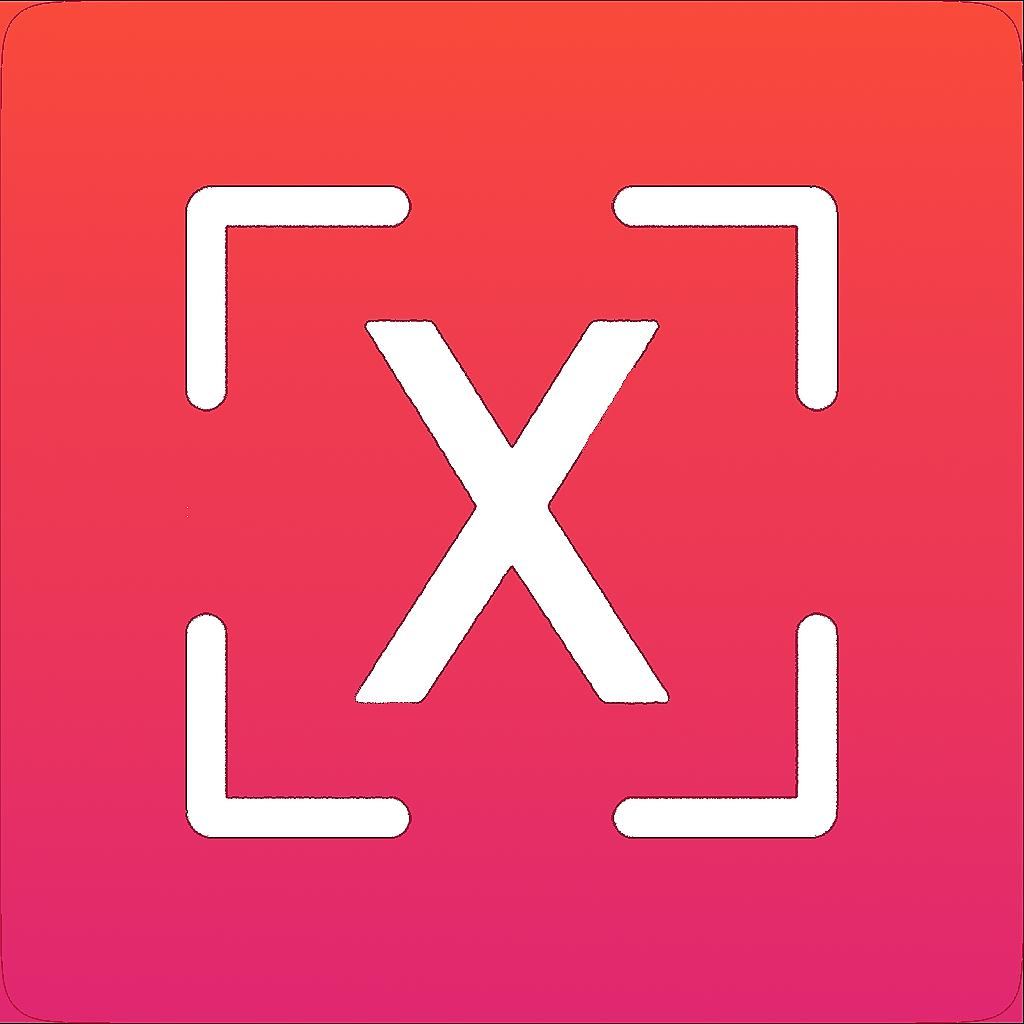Math Box - Math Answer Scanner-Math Solver & Homework Helper 4 8279

"mathstudio" has 207 apps. Top 5 are MathStudio, Mathway: Math Problem Solver, Microsoft Math Solver - HW app, MathStudio Express, and Math Box - Math Answer Scanner.

• matlab graphing calculator  Related Apps & App Analytics
 Apps Average Star RatingsMathStudio-Symbolic graphing calculator 4 135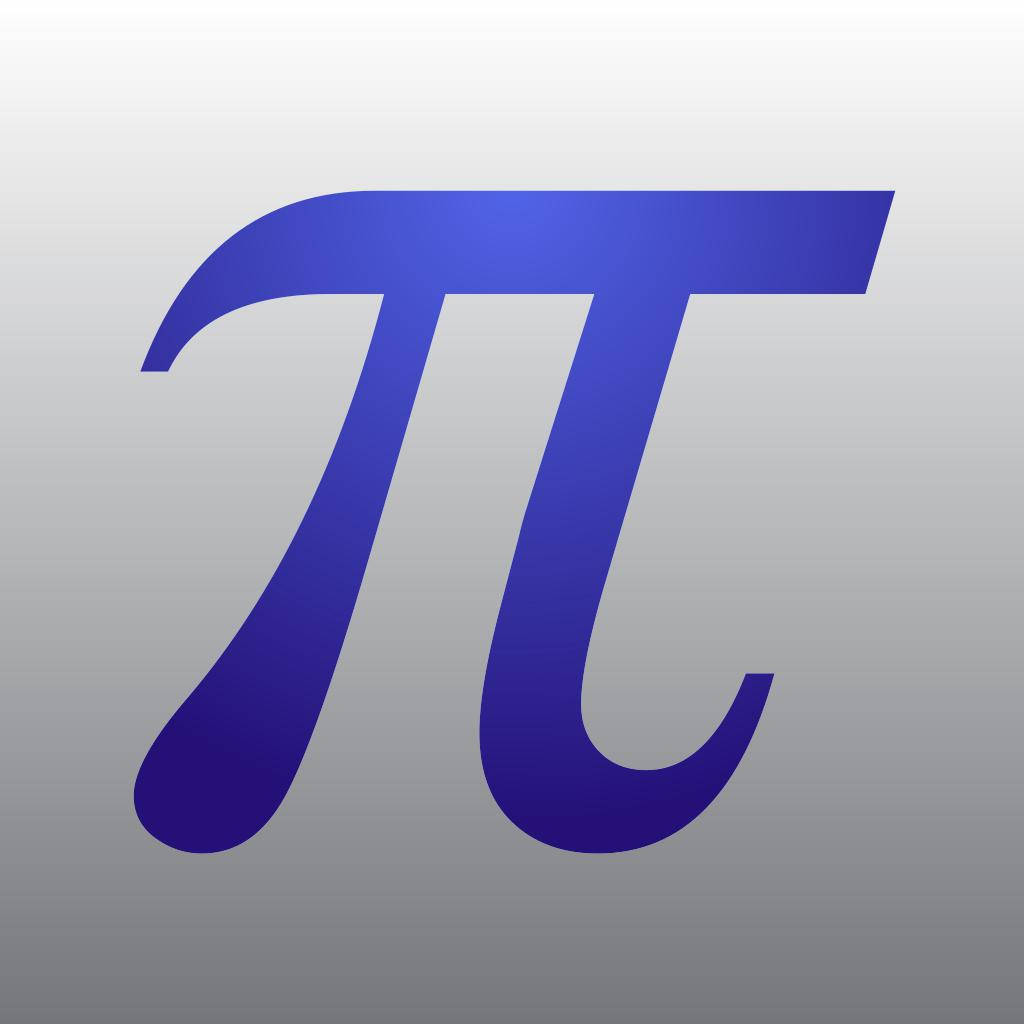Mathematics with PocketCAS Pro-Graphing, Calculus and Algebra 4 393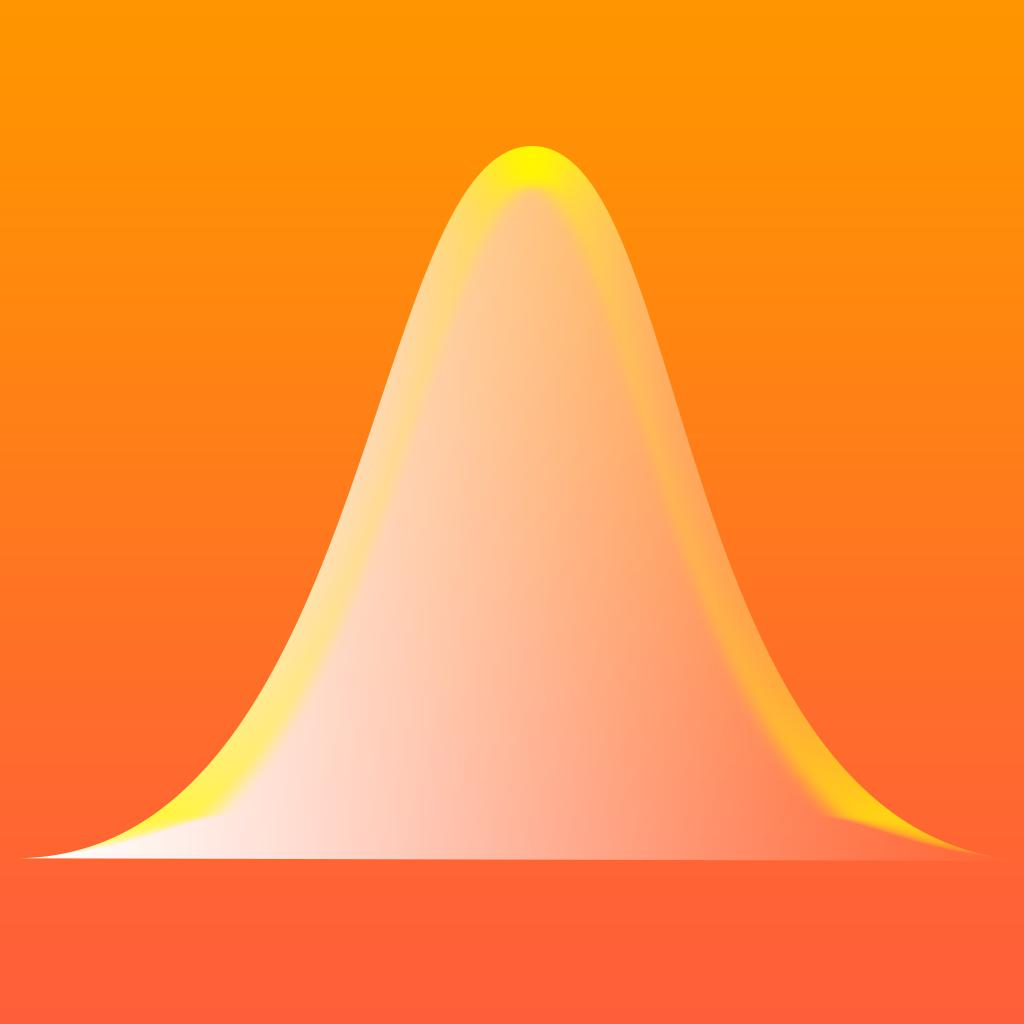Bell Curves - graphing calculator for the normal distribution function 3 2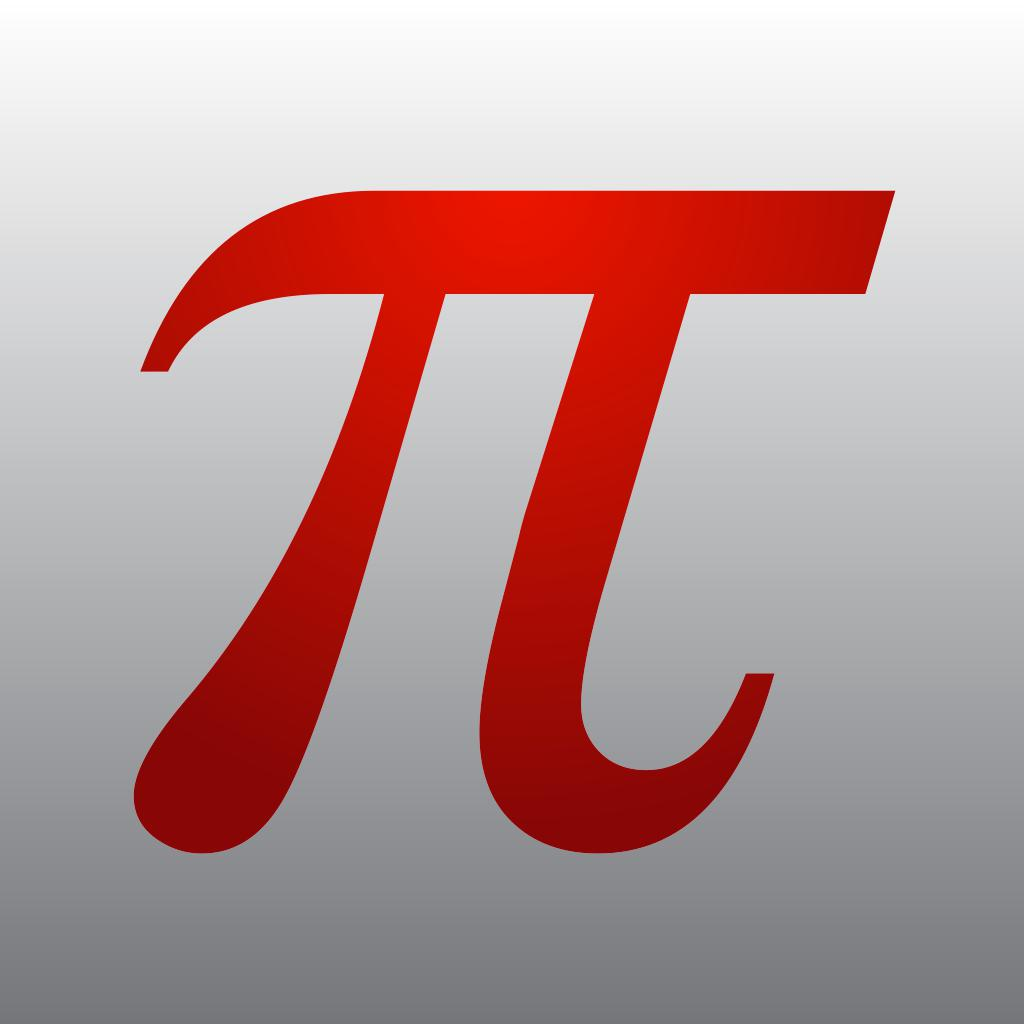PocketCAS lite for Mathematics-Helps with Calculus + Algebra 4 114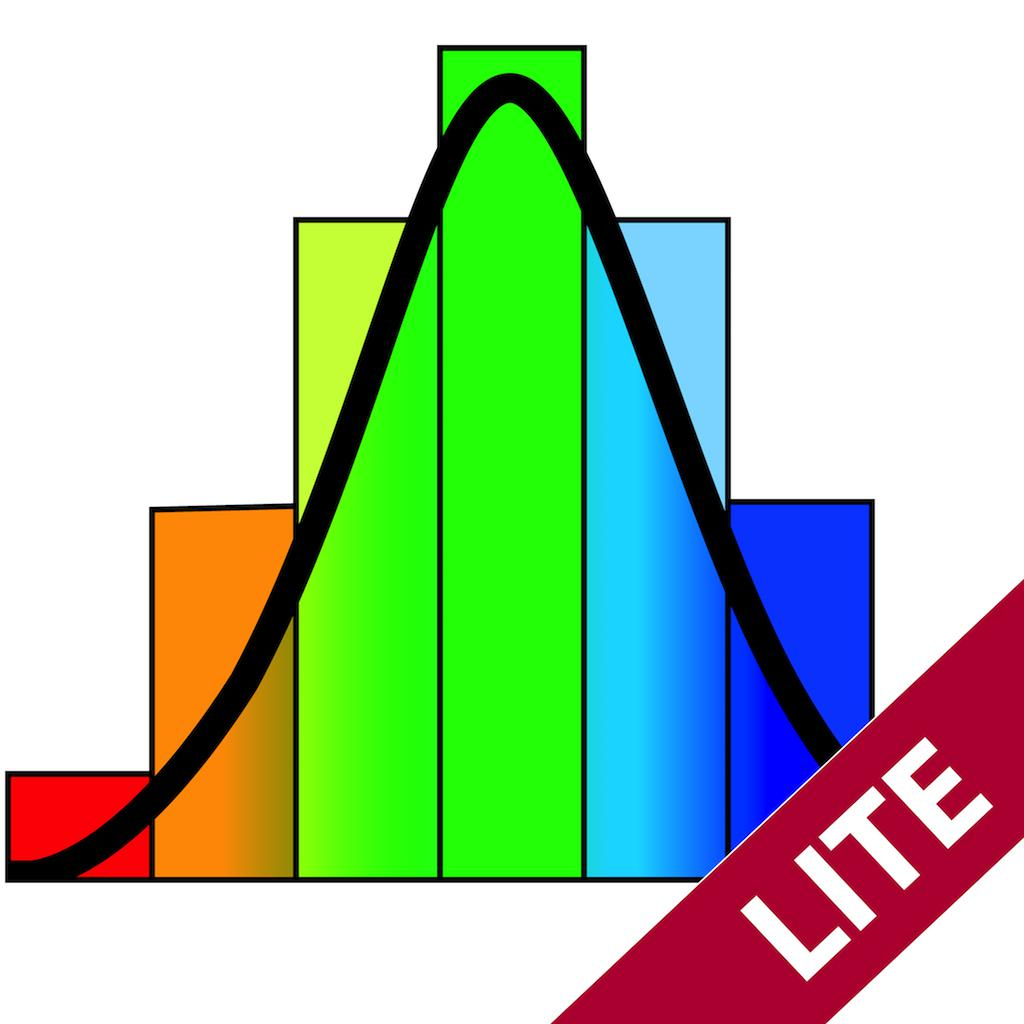Math Graphing Lite - Dynamic Scientific Calculator 4 3

"matlab graphing calculator" has 6 related apps in total. Top 5 are MathStudio, Mathematics with PocketCAS Pro, Bell Curves - graphing calculator for the normal distribution function, PocketCAS lite for Mathematics, and Math Graphing Lite - Dynamic Scientific Calculator.

• math studio  Related Apps & App Analytics
 Apps Average Star RatingsMathStudio-Symbolic graphing calculator 4 135Mathway: Math Problem Solver-Homework scanner & calculator 5 309194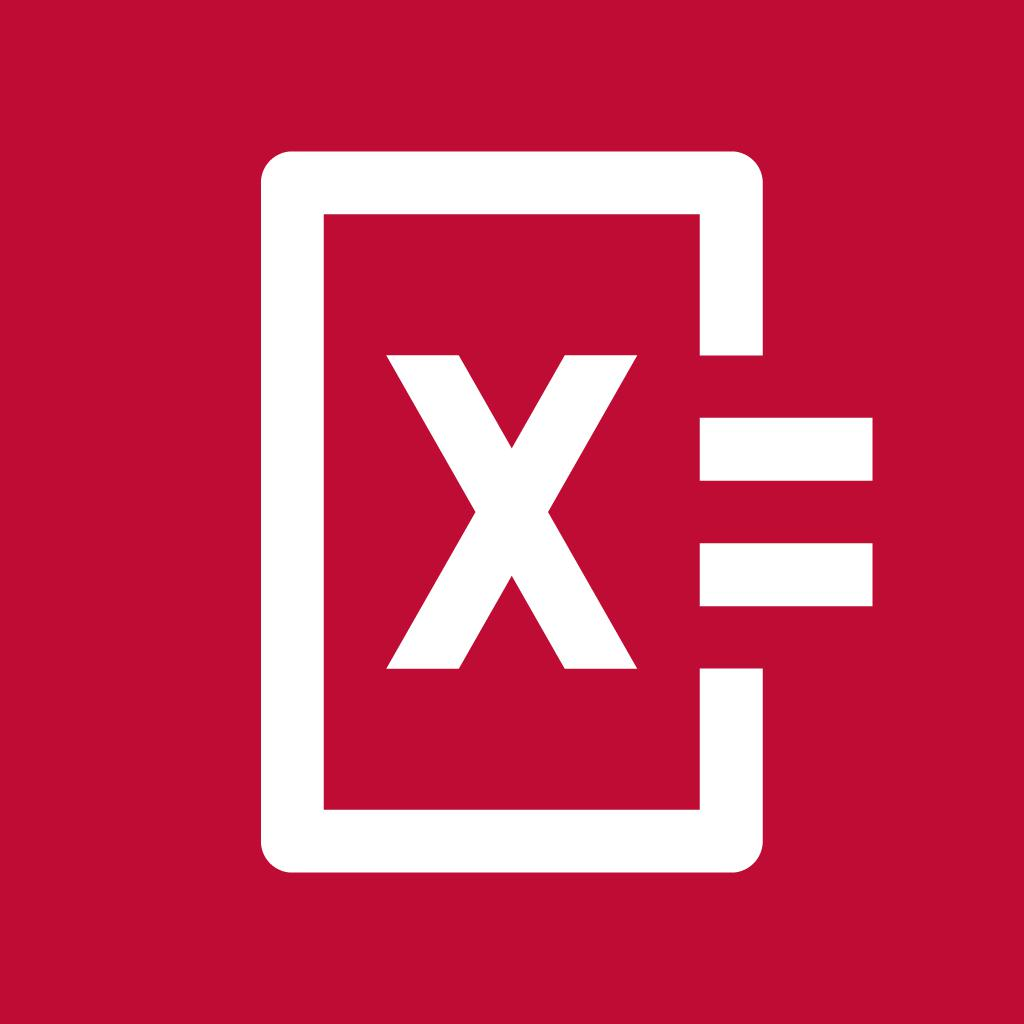Photomath-Math explained, step-by-step 5 456568Elevate - Brain Training-Award-winning brain games 5 282242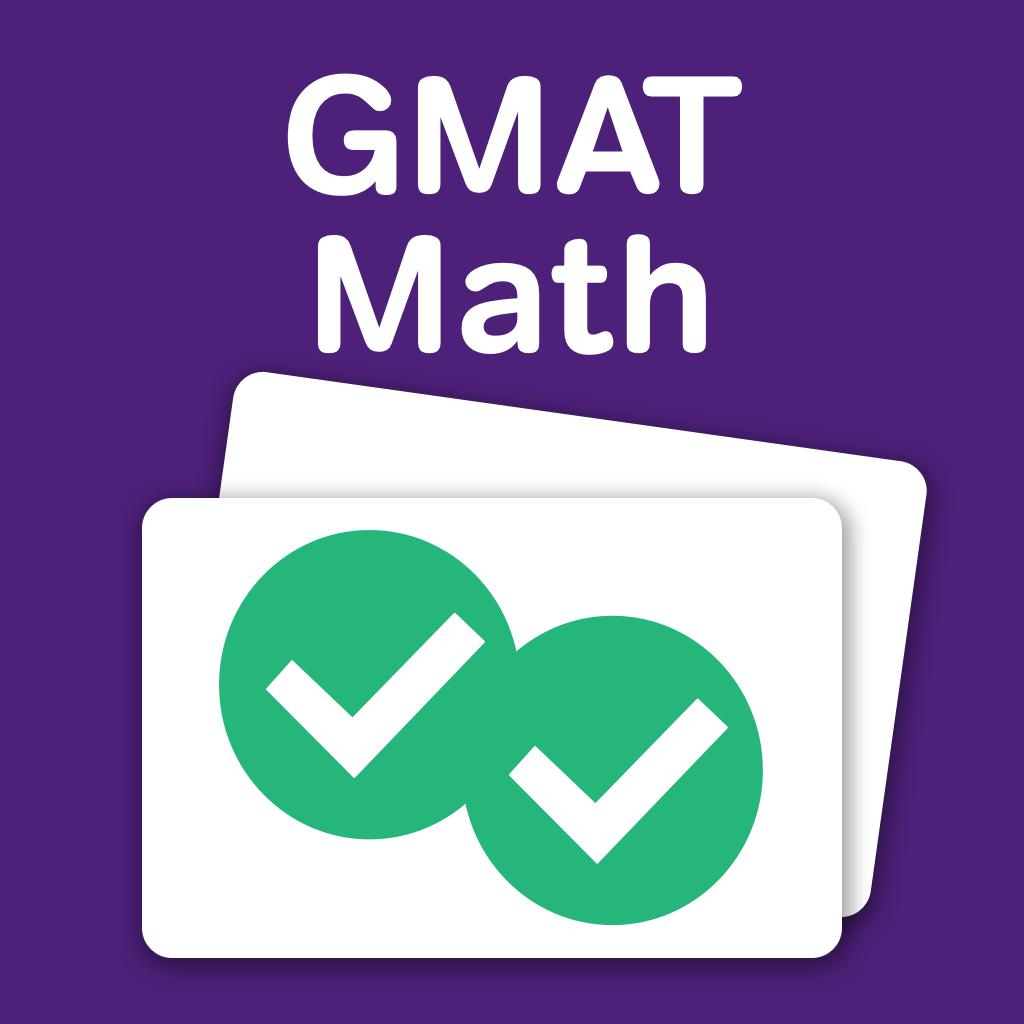GMAT Math Flashcards-Magoosh 5 1196

"math studio" has 229 related apps in app store. Top 5 are MathStudio, Mathway: Math Problem Solver, Photomath, Elevate - Brain Training, and GMAT Math Flashcards.

• pomegranate apps llc  Related Apps & App Analytics
 Apps Average Star RatingsNutrients - Nutrition Facts-Recipe Manager & Food Journal 4 654MathStudio-Symbolic graphing calculator 4 135MathStudio Express-Symbolic graphing calculator 4 84Solve - Graphing Calculator-A colorful graphing calculator 5 159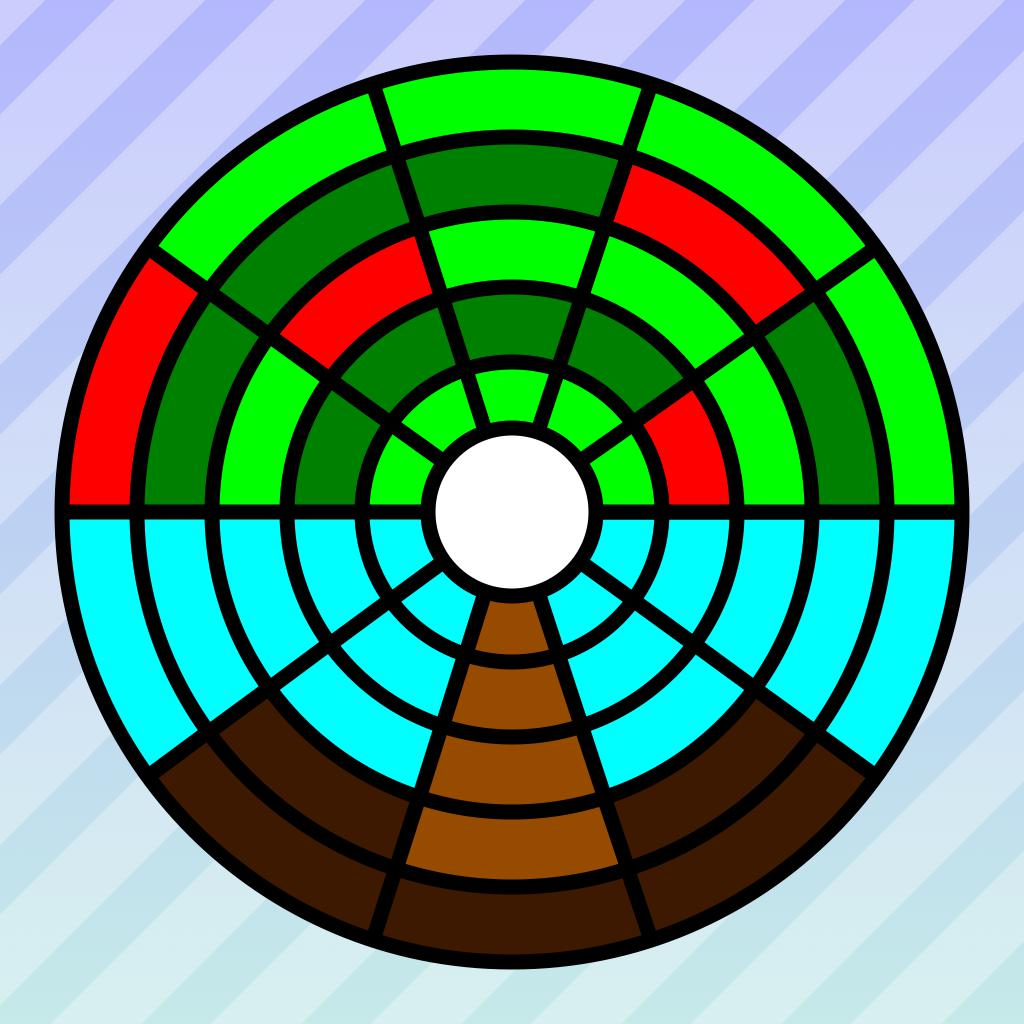Arcs-Circular puzzle game 5 47

"pomegranate apps llc" has 20 related apps. Top 5 apps are Nutrients - Nutrition Facts, MathStudio, MathStudio Express, Solve - Graphing Calculator, and Arcs.

• ارائه مطلب  Related Apps & App Analytics
 Apps Average Star RatingsPocketCAS lite for Mathematics-Helps with Calculus + Algebra 4 114Mathematics with PocketCAS Pro-Graphing, Calculus and Algebra 4 393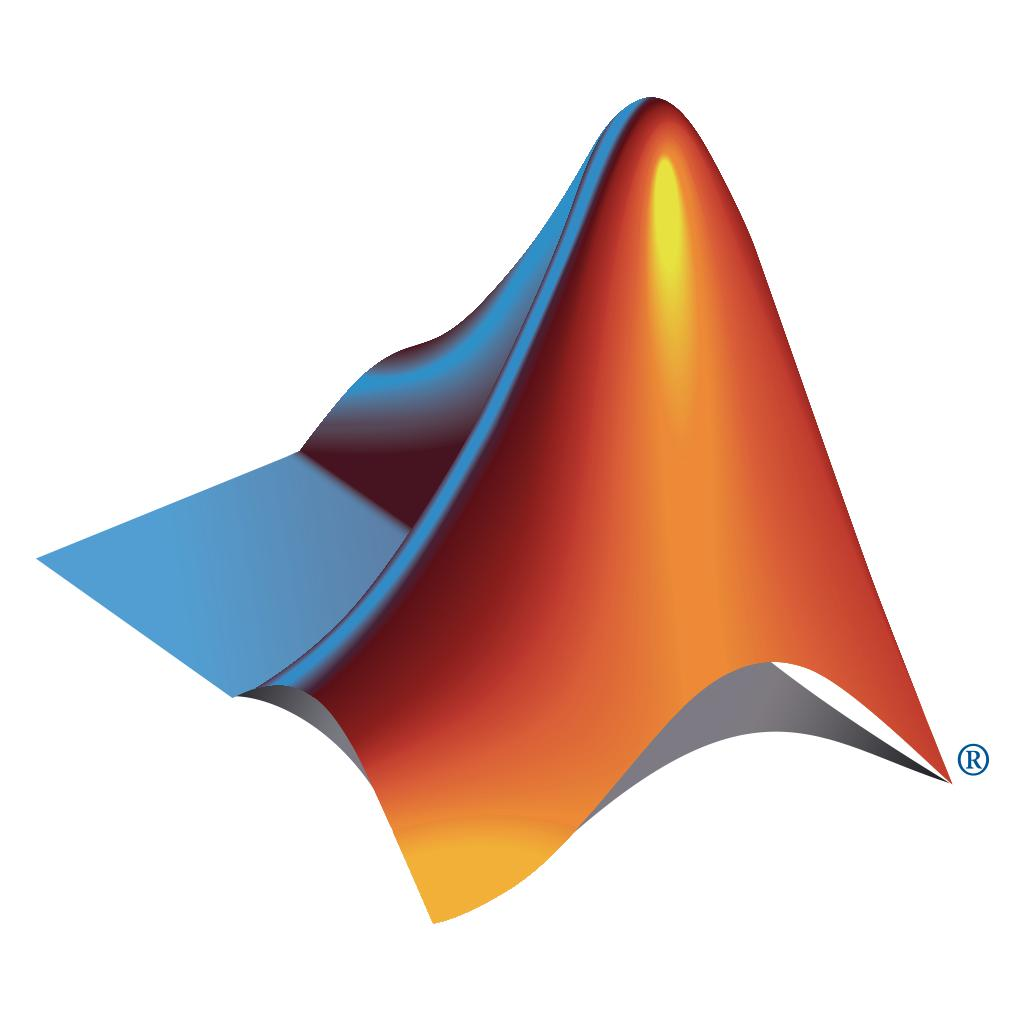MATLAB Mobile 3 103MathStudio-Symbolic graphing calculator 4 135العاب ورقيه سحريه 3 10

66 apps are ranking for "ارائه مطلب" in app store. Top 5 apps are PocketCAS lite for Mathematics, Mathematics with PocketCAS Pro, MATLAB Mobile, MathStudio, and العاب ورقيه سحريه.

As you can see, MathStudio, MathStudio, and Mathway: Math Problem Solver are ranking for mathstudio, also, MathStudio, MathStudio, Mathematics with PocketCAS Pro and PocketCAS lite for Mathematics are all competing the keyword matlab graphing calculator.

Before promoting your apps in app store, you'd better perform app analytics for better ASO tactics, and ASOTools, free ASO tools will help you with App store keywords optimization.